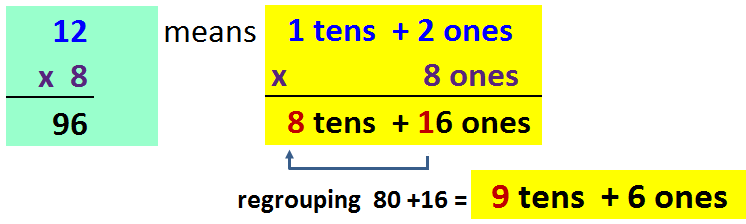# Multiply

## Multiplying whole numbers

Multiplication is a mathematical operation that indicates how many times a number is added to itself.

2 x 5 = 10                                        5 + 5 = 10

Multiplying two groups of 5           Adding two groups of 52 x 5 = 10

2 x 5 = 2 + 2 + 2 + 2 + 2 = 10Multiplicand is a number that is to be multiplied by another (the multiplier). Product is the result of multiplying two (or more) numbers, for instance, 10 is the product of 2 and 5.

###Factors are the numbers that are multiplied together.

In this method we multiply the multiplicand by each digit of the multiplier and then add up all the properly shifted results.Steps:

Step one: Multiply by ones digit to get the first partial product.

Step two: Multiply by tens digit to get the second partial product.

Step three: Multiply by hundreds digit to get the third partial product.

Step four: …

Last step: Add all partial products together to get the final product!

Example:

In this example, we multiply by the units and the tens separately.34 x 21 = 34 x (20 + 1) = 34 x 20 + 34 x1 =680 + 34 = 714

The box method (partial products method) works by splitting each number into tens and units

Example:   34 = 30+4  and   21 = 20+134 x 21 = 600+80+30+4 = 714### The Distributive Property### IN08     Multiplying and Dividing Whole Numbers

Word Problems – Using Whole Numbers

### WN45     Multiplying Wole Numbers by multiples of 10